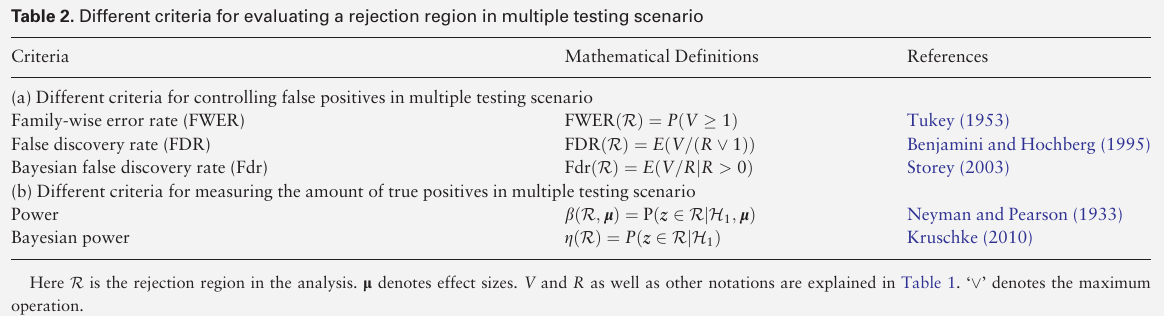# Joint Local False Discovery Rate in GWAS

##### Posted on Nov 12, 2022 (Update: Nov 14, 2022)
$\newcommand\Jlfdr{\mathrm{Jlfdr}} \newcommand\Fdr{\mathrm{Fdr}}$

propose a novel summary-statistics-based joint analysis method based on controlling the joint local false discovery rate (Jlfdr).

• prove that the method is the most powerful summary-statistics-based joint analysis method when controlling the false discovery rate at a certain level
• the Jlfdr-based method achieves higher power than commonly used meta-analysis methods when analyzing heterogeneous datasets from multiple GWASs

two kinds of joint analysis methods:

• individual-level
• summary-statistics-based

Jlfdr generalizes the concept of the local false discovery rate (Jlfdr) from the analysis of single study to the joint analysis of multiple studies## Methods

### Jlfdr and optimal rejection region

$\Jlfdr(z) = P(H_0\mid \bfz)$

Fdr is the expectation of Jlfdr, given that the test statistic vector is in the rejection region $R$.

$\Fdr(R) = E(\Jlfdr(z)\mid z\in R)$

### Implementation of Jlfdr-based method under the Gaussian mixture model

Published in categories Note